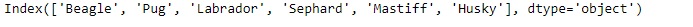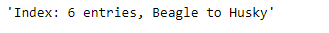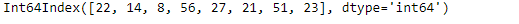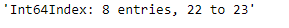Related Articles
Python | Pandas Index.summary()
• Last Updated : 18 Dec, 2018

Python is a great language for doing data analysis, primarily because of the fantastic ecosystem of data-centric python packages. Pandas is one of those packages and makes importing and analyzing data much easier.

Pandas` Index.summary()` function return a summarized representation of the Index. This function is similar to what we have for the dataframes.

Syntax: Index.summary(name=None)

Returns : Summary

Example #1: Use `Index.summary()` function to find the summary of the Index.

 `# importing pandas as pd``import` `pandas as pd`` ` `# Creating the index ``idx ``=` `pd.Index([``'Beagle'``, ``'Pug'``, ``'Labrador'``,``                ``'Sephard'``, ``'Mastiff'``, ``'Husky'``])`` ` `# Print the index``idx`

Output :Now we will find the summary of the Index.

 `# find the summary of the Index.``idx.summary()`

Output :As we can see in the output, the function has returned an overall summary of the Index.

Example #2: Use `Index.summary()` function to summarize the Index.

 `# importing pandas as pd``import` `pandas as pd`` ` `# Creating the index ``idx ``=` `pd.Index([``22``, ``14``, ``8``, ``56``, ``27``, ``21``, ``51``, ``23``])`` ` `# Print the index``idx`

Output :Now we will find the summary of the index.

 `# the function returns the summary of the Index``idx.summary()`

Output :As we can see in the output, the function has returned the summary for the Index.

Attention geek! Strengthen your foundations with the Python Programming Foundation Course and learn the basics.

To begin with, your interview preparations Enhance your Data Structures concepts with the Python DS Course. And to begin with your Machine Learning Journey, join the Machine Learning – Basic Level Course

My Personal Notes arrow_drop_up